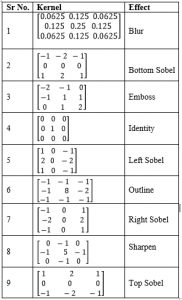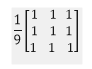Dulari Bhatt — Published On August 8, 2021 and Last Modified On August 8th, 2021

This article was published as a part of the Data Science Blogathon

## Introduction to Convolution

Convolution is a trendy term in computer vision (CV). When discussing how to implement any CV task, CNN, or Convolutional Neural Network, is frequently mentioned. As a result, any CV aspirant must comprehend the phrase “Convolution fully.”

Convolution is a basic mathematical operation that several image processing operators use. Convolution is a method of multiplying two arrays of integers, often of different sizes but of the same dimensionality, to produce a third array of the same dimensionality.

Application of convolution includes signal processing, statistics, probability, engineering, physics, computer vision, image processing, acoustics, and many more. Image processing includes image filtering, noise removal, image recognition, image segmentation, etc. Convolution has a great role to play in image filtering.

## Image filtering with convolution

Image filtering is changing the pixel value of a specific image to blur, sharpen, emboss, or make edges more clear. It changes the appearance of the original image. Nowadays many image filters are ruling social media applications.  Most people, using Instagram, maybe knowing about the image filters used in it.  Image filtering is application-specific, as sometimes we need to blur the image and sometimes we wish to have sharpness in the image.

It is very easy to use and see the filters on our image. It is very fascinating to learn the maths and image processing behind it. Image filters use various convolution kernels to perform different image filters.

Convolution filters, sometimes known as kernels, are used with images to achieve blurring, sharpening, embossing, edge detection, and other effects. This is performed through the convolution of a kernel and an image. Kernels are typically 3×3 matrices, and the convolution process is formally described as follows:

g(x,y)=w*f(x,y)

Where g(x,y) represents the filtered output image, f(x,y) represents the original image, and w represents the filter kernel. The graphic below shows how the convolution works.

[Explanation of convolution process ]

Thus, the above image clearly shows that the output will be changed if we change the kernel. There are many convolutional kernels for sharpening, blurring, or smoothing the image. The following table displays various kernels and its effect.[Table1. Various kernels and their effect]

## Implementation of Convolution for various image filtering.

### 1. Box Blur

The box blur is a straightforward blur in which each pixel is set to the average of the pixels surrounding it. It may be written as a discrete convolution of two functions f[n] and g[n], where f[n] represents the image’s discrete pixel values and g[n] is our kernel, which is a matrix denoted as[Fig 1. Box blur filter]

## Implementation steps:

### Step1: Open Google colab notebook

Google colab was used for implementation. Colaboratory, or “Colab” for short, enables you to write and run Python in your browser with

•  No configuration is necessary
•  Simple sharing

Colab can help you whether you’re a student, a data scientist, or an AI researcher. To get started with Google Colab, search for “google colab” and click on the first link. Then select “create a new notebook.” You are now ready to begin writing code.

### Step 2: Import libraries

```import cv2
import numpy as np
import matplotlib.pyplot as plt```

### Step 3: Reading an image and plotting it

```image = cv2.imread('/content/1.PNG')
image = cv2.cvtColor(image, cv2.COLOR_BGR2RGB)
fig, ax = plt.subplots(1, figsize=(12,8))
plt.imshow(image)```

output:

### Step4: Make the Kernel and apply convolution

let’s make a kernel
for blurring the image. We will use Numpy to build a 3×3 matrix of ones, and
divide it by 9, as shown in Fig.1. filter2D() function gives the convolution of the input array and kernel.

```abc=np.ones((3,3))
kernel = np.ones((3, 3), np.float32) / 9
img = cv2.filter2D(image, -1, kernel)
fig, ax = plt.subplots(1,2,figsize=(10,6))
ax.imshow(image)
ax.imshow(img)```

The output of Box Blur:

## 2. Sharpening

Image sharpening is used to sharpen the image. There are three main reasons behind image sharpening:

1.  To overcome blurring introduced by the camera,
2.  To draw attention to particular areas
3.  To increase legibility.

Any current camera’s RAW data are always slightly unsharp. Blur is introduced at every stage of the image capture process. First, some definition is lost as the light goes through the lens elements, no matter how finely crafted. Second, the sharpest transitions are averaged out and slightly blurred when the sensor processes the photons that fall on it. Finally, a modest blur is introduced when the three different colour channels are interpolated to create the final image.

Second, contrast attracts human eyes. When we look at a photograph, our attention is drawn to the finest elements. So, if you want to direct a viewer’s attention, selective sharpening is one of the greatest techniques to accomplish so.

Finally, enhancing an image improves the visibility of essential elements. For example, the text becomes easier to read, individual leaves become more visible, and faces in a throng become more distinct. Sharpening is done with the kernel below.

## Implementation

Following is the code for having sharpening in the image,

```import cv2
import numpy as np
import matplotlib.pyplot as plt
image = cv2.cvtColor(image, cv2.COLOR_BGR2RGB)
kernel = np.array([[0, -1, 0],
[-1, 5, -1],
[0, -1, 0]])
img = cv2.filter2D(image, -1, kernel)
fig, ax = plt.subplots(1,2,figsize=(10,6))
ax.imshow(image)
ax.imshow(img)```

The output of Sharpen:

## 3. Emboss

Emboss means to form a mould a 3D mould that sticks out from the surface. When an emboss filter is applied to a picture, the resulting image resembles an emboss – a paper or metal emboss of the original image in which the features stand out in high relief (more prominently) or low relief (less prominently) (less prominently). Following is the convolution kernel for image embossing…

## Implementation

For implementing image embossing, we have to
follow the same procedure as explained in box blur. The only change is in its
kernel.

```import cv2
import numpy as np
import matplotlib.pyplot as plt
image = cv2.cvtColor(image, cv2.COLOR_BGR2RGB)
kernel = np.array([[-2, -1, 0],
[-1, 1, 1],
[0, 1, 2]])
img = cv2.filter2D(image, -1, kernel)
fig, ax = plt.subplots(1,2,figsize=(10,6))
ax.imshow(image)
ax.imshow(img)```

The outputt of embossing:

Thus, as shown in table1, you can change the kernel, and accordingly, you can have various image filters.

## Concluding remarks:

Convolution is nothing but a simple mathematical function, which is used for various image filtering techniques. Convolution uses a 2input matrix: that is, image matrix and kernel. With the help of that, by performing convolution, it generates the output. As you change the kernel, you can also notice the change in the output.

## Reference

1. http://cse3521.artifice.cc/images/3d_convolution_animation.gif

2. https://setosa.io/ev/image-kernels/

3. https://www.sciencedirect.com/topics/computer-science/convolution-filter